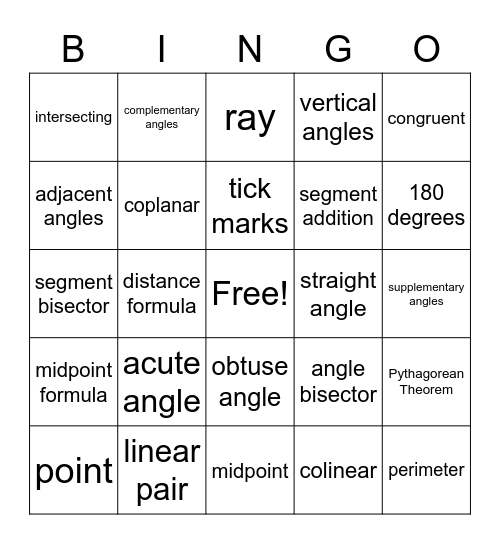Geometry Tools BingoThis bingo card has a free space and 29 words: point, 90 degrees, distance formula, line, intersecting, ray, coplanar, acute angle, straight angle, linear pair, supplementary angles, perimeter, midpoint, colinear, 180 degrees, area, obtuse angle, midpoint formula, congruent, segment bisector, angle bisector, plane, segment addition, adjacent angles, vertical angles, complementary angles, Pythagorean Theorem, tick marks and congruent angles.

Play Online